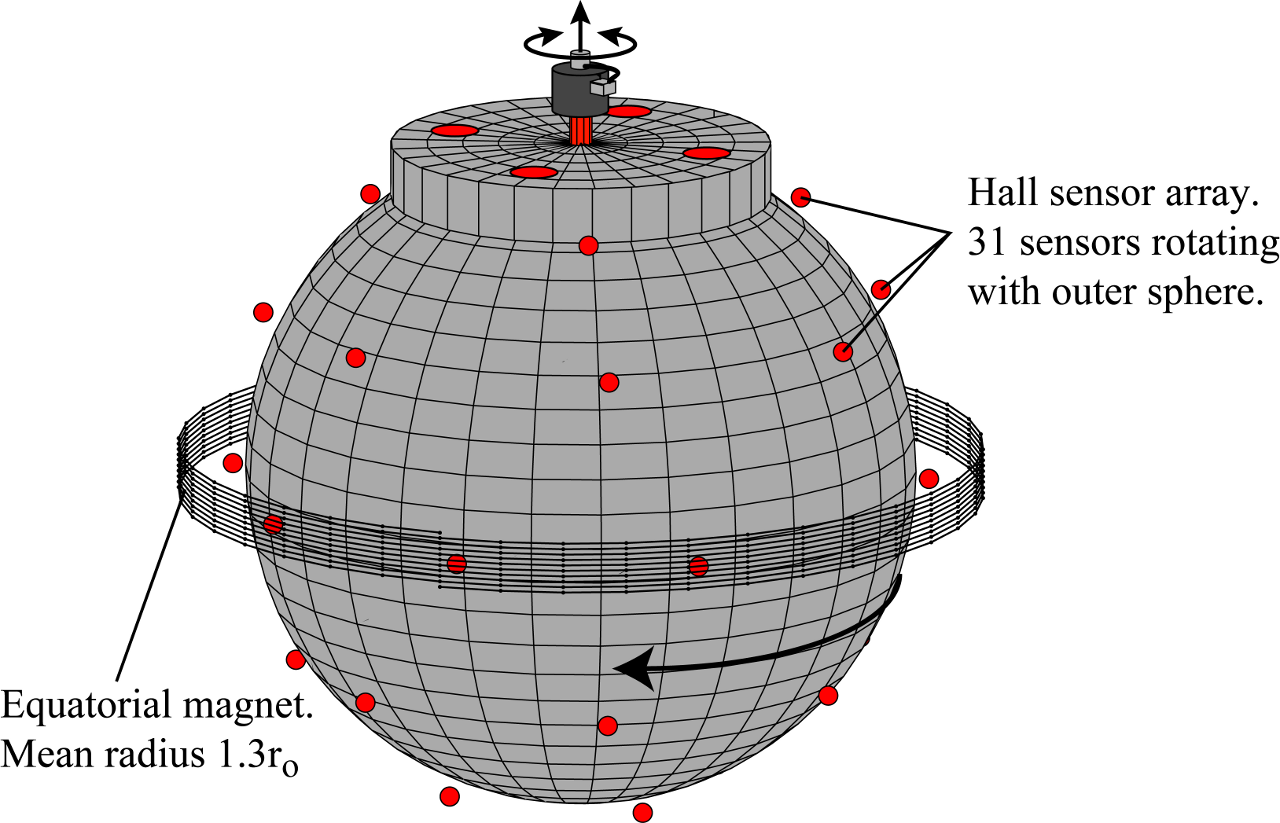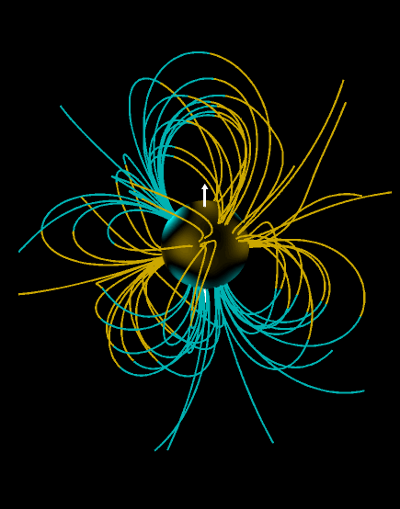#### Magnetic Field Visualization Code

Since we're trying to understand how a turbulent liquid metal flow interacts with magnetic field, the time-varying magnetic field outside the three meter experiment is one of the most important measurements. At present, we measure the spherical radial component of the magnetic field just outside the non-magnetic stainless steel shell. In experiments so far, we have not generated a magnetic field, but a large computer-controlled electromagnet in the equatorial plane (sketched below) can be used to apply a field to see how it can interact with the turbulence.Hall effect sensor array schematic.

We model the external field using a set of vector spherical harmonics up to degree and order four. We fit the measurements from the 31 probes to this field model and retrieve time series of coefficients for each spherical harmonic component of the field. Since we're measuring field in the air around the sphere where no currents are flowing, we can use this model field to reconstruct all three vector components of the magnetic field anywhere outside the experiment and use that to draw field lines for nice visualizations of the magnetic state.Magnetic field snapshot, Ro = +1.5, Λ=0.05

A single snapshot of induced magnetic field for the state is shown above. The radial field on the surface of the sphere is shown as brightness of color on a sphere with yellow and blue representing north and south magnetic polarity. Field lines around the sphere are drawn colored according to polarity as well. The main field line calculation code takes an instantaneous snapshot of the model field coefficients, generally known as Gauss coefficients in geomagnetic practice, and integrates lines (using the Euler-Cromer method) from a chosen set of inital points. The code is in MATLAB and is vectorized over field lines for speed. The main code for line calculation is bline_spag.m at https://github.com/danzimmerman/matlabmag. (If you wish to use this code, please check the README and note that there are several dependencies, like gm3cart.m)

The time series of Gauss coefficients along with this code can be used to generate movies of the time-dependent magnetic field. Shown below is a movie of a newly discovered rotating wave that arises for strong counter-rotation of the inner sphere and strong applied magnetic field.

Magnetic Field Movie, Ro=-4.3, Λ=0.43, m=2 Rotating Wave